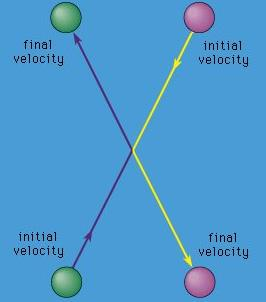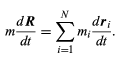Courses

# Relative motion Physics Notes | EduRev

## Physics : Relative motion Physics Notes | EduRev

The document Relative motion Physics Notes | EduRev is a part of the Physics Course Basic Physics for IIT JAM.
All you need of Physics at this link: Physics

A collision between two bodies can always be described in a frame of reference in which the total momentum is zero. This is the centre-of-mass(or centre-of-momentum) frame mentioned earlier. Then, for example, in the collision between two bodies of the same mass discussed above, the two bodies always have equal and opposite velocities, as shown in Figure. It should be noted that, in this frame of reference, the outgoing momenta are anti parallel and not perpendicular.Figure 14: Collision between two particles of equal mass as seen from the centre-of-mass frame of reference.

Any collection of bodies may similarly be described in a frame of reference in which the total momentum is zero. This frame is simply the one in which the centre of mass is at rest. This fact is easily seen by differentiating equation with respect to time, giving(70)

The right-hand side is the sum of the momenta of all the bodies. It is equal to zero if the velocity of the centre of mass, dR/dt, is equal to zero.
If Newton’s second law is correct in any frame of reference, it will also appear to be correct to an observer moving with any constant velocity with respect to that frame. This principle, called the principle of Galilean relativity, is true because, to the moving observer, the same constant velocity seems to have been added to the velocity of every particle in the system. This change does not affect the accelerations of the particles (since the added velocity is constant, not accelerated) and therefore does not change the apparent force (mass times acceleration) acting on each particle. That is why it is permissible to describe a problem from the centre-of-momentum frame (provided that the centre of mass is not accelerated) or from any other frame moving at constant velocity with respect to it.
If this principle is strictly correct, the fundamental forces of physics should not contain any particular speed. This must be true because the speed of any object will be different to observers in different but equally good frames of reference, but the force should always be the same. It turns out, according to the theory of James Clerk Maxwell, that there is an intrinsic speed in the force laws of electricity and magnetism: the speed of light appears in the forces between electric charges and between magnetic poles. This discrepancy was ultimately resolved by Albert Einstein’s special theory of relativity. According to the special theory of relativity, Newtonian mechanics breaks down when the relative speed between particles approaches the speed of light (see the article relativistic mechanics).

Offer running on EduRev: Apply code STAYHOME200 to get INR 200 off on our premium plan EduRev Infinity!

,

,

,

,

,

,

,

,

,

,

,

,

,

,

,

,

,

,

,

,

,

;